(Version >= 3.98)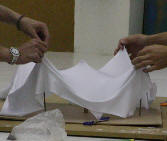We have already mentioned that the process the Mesh menu is the same as the modeling method, i.e. modifying the form of a deformable membrane, like with a Lycra membrane as shown in the picture. We assume that we have a very large piece of lycra. According to the model that we try to construct, we cut this piece of Lycra with a certain perimeter.

This is precisely what we do with the WinTess3 program.

Entering the coordinates of the vertices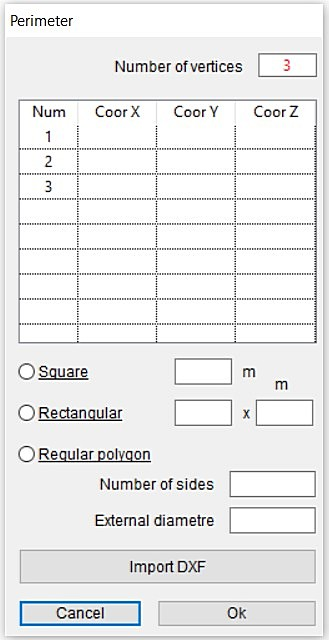When using the mesh menu, the first thing that appears is the window for the perimeter of virtual fabric which we are going to cut. This perimeter may be in very different ways:

• Manual entry
First we set the number of vertices. Later we carefully enter X, Y, Z coordinates of these vertices in counter clockwise way. Although we can only use X and Y for virtual fabric trimming, the Z-coordinate will be useful in subsequent processes in the main window of the program.
• Square mesh
When the perimeter is a square, we only have to enter the side of the square in m.
• Rectangular mesh
In this case, we must enter two values: the length and the width of the virtual fabric in m.
• Regular poligon
First we introduce the number of sides (equal to the number of vertices) of the polygon and then the length of the diameter of the circle circumscribed the polygon in m.
• Import DXF
Finally, there is the option to import a perimeter from a DXF type file (any CAD program uses this type of files). We must keep in mind that the program imports only a single closed polyline (2D or 3D) located on a layer called WINTESS. The coordinates of the CAD file have to be in m. Just like the previous cases, the X,Y coordinates will be used to cut the virtual fabric.

Except the first choice where the coordinates are entered by hand, the coordinates in other cases are automatically generated depending on the type of perimeter that we used.

Irregular mesh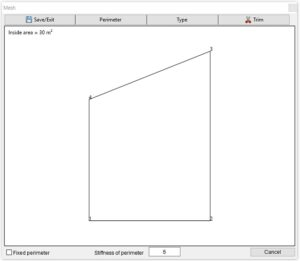At any time, we can modify the coordinates in the table whether they are entered by hand, or generated automatically. This way, we may generate an irregular perimeter using the automatic coordinates of a regular polygon.

During the creation of the mesh, we simultaneously see the perimeter graphic and its internal area (m²) in the main window.

Once delimited with the perimeter, we generate a mesh that represents the membrane with which we are going to work. The best type of mesh representing the membrane is not something definite. There are infinite number of meshes representing the same membrane. Although this infinite number, only a few have properties related to the membrane. We refer to the following regular meshes: triangular, square, rectangular and radial.

Fixed perimeter

If we want a mesh with fixed perimeter, at this stage, we can indicate it by checking the box that appears at the bottom of the screen: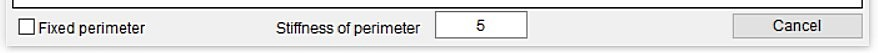Likewise, at any time, we can also exit the process of mesh creation using the Cancel button at the very bottom.

Mesh type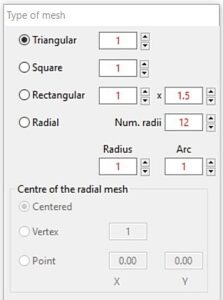A mesh is defined by elements (bars) of the mesh. If we are talking about a regular mesh, a triangular mesh is one in which the bars are equilateral triangles. Similarly a square and rectangular meshes are the ones with squares and rectangles, respectively.

It is necessary to define the size of the mesh base by providing a value (m) for the side of the triangle or square meshes, and two values for sides of rectangular meshes. This size is going to determine the mesh density.

Radial meshes are formed by intersection of a set of bars that depart from a point (center) in a uniform radial form with another set of bars that are circular concentric (the center which we have mentioned above). They form some isosceles triangles touching at the center and also a few isosceles trapezoids in the rest of the mesh.

In the case of a radial mesh, a singular point is very important: the center. The user must define the coordinates of that point, either directly or indirectly. In the latter case, it may refer to the center of the window where the perimeter is drawn or to one of the vertices of the perimeter.

Mesh is defined by the number of radial lines (num. radii), the distance between concentric circles (radius) and the maximum length of the arc to divide each of these circles (arc). When the arc length exceeds the supplied value, the number of radials doubled and the process begins again.

Mesh examples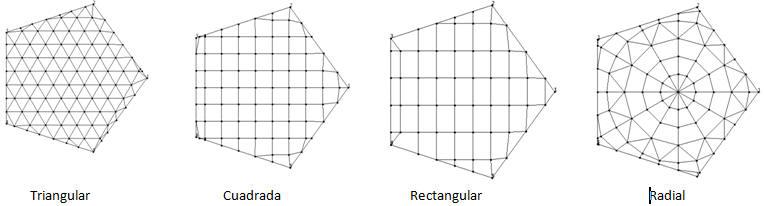Changing position of the mesh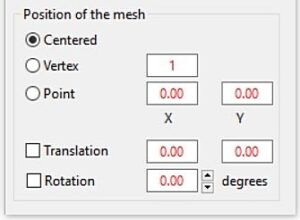In the above image, we see examples of meshes already cut off by perimeter. But before cutting the mesh, the program allows you to modify them a bit. For example, suppose that we have a square mesh on a pentagonal perimeter.
Before we can cut:

• Center the mesh. This is the default option.
• The mesh passes through a selected vertex.
• The mesh passes through a point P with defined x,y coordinates
• The mesh moves an increment of X,Y (dx,dy)
• The mesh turns counter-clockwise by an angle in degrees

In the figure given below, we have moved the mesh to pass through the vertex 1 which was more on the right.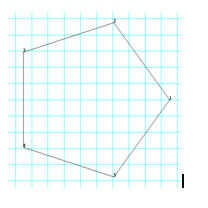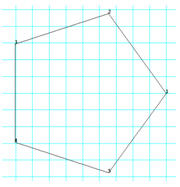Trim and finish

The main window, which we discussed previously, has the following menu:

• Save/Exit
As its name suggests, it is used to finish the stage of mesh creation.
If we are satisfied with the generated mesh, WinTess will keep it in a file.
Otherwise, it will not be saved and we continue doing something else with the program. NOTE: The generated mesh is flat. It is on X,Y plane. However, the corners of perimeter have three coordinates X, Y, Z that we have assigned. We see the third dimension when the mesh is displayed in 3D screen.
• Perimeter
At any time, we can go back to the perimeter window to modify it if we see some error.
• Type
It opens the window of mesh types to change the current or to modify if necessary.
• Trim
This button cuts off the mesh inside of the perimeter and modifies the bars on the perimeter.